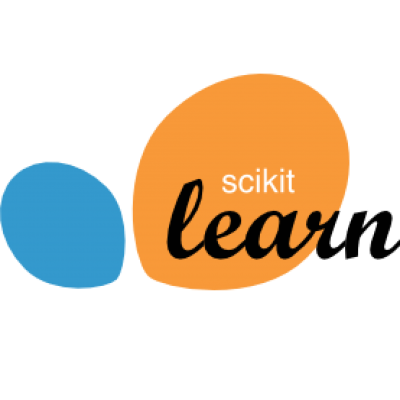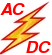RR, SO Random Reshufflig and Shuffle Once algorithms. Based on the paper Random Reshuffling: Simple Analysis with Vast Improvements, arXiv:2006.05988, 2020. SAGA-AS SAGA with arbitrary sampling. Based on this ICML 2019 paper. REX (R Shiny) Randomized EXchange algorithms, implemented as web-based R Shiny apps, for computing optimal design of experiments and minimum volume enclosing ellipsoids. Based on this paper. SPDHG Stochastic Chambolle-Pock method (aka: Stochastic Primal-Dual Hybrid Gradient method). Based on this paper. See also this follow-up paper with application to PET imaging. StochBFGS Stochastic (block) BFGS method for solving the empirical risk minimization problem with  logistic loss and L2 regularizer. Related paper. Random Inverse A suite of randomized methods for inverting positive definite matrices implemented in MATLAB. Related paper. Random Linear Lab A lab for testing and comparing randomized methods for solving linear systems. Implemented in MATLAB. Related paper. CoCoA A framework for communication-efficient distributed optimization for machine learning.Accelerated, Parallel and PROXimal coordinate descent. This is an efficient C++ code based on this paper. We also implement PCDM (parallel coordinate descent), SDCA (stochastic dual coordinate ascent) and AGD (Accelerated Gradient Descent). APPROX is implemented in. Choose option "accelerated" when doing LASSO/elastic net. S2GD Semi-stochastic gradient descent method for fast training of L2 regularized logistic regression. This is an efficient C++ code (can be called from MATLAB), based on this paper.Parallel Sparse PCA [8 9] code. Supports multicore workstations, GPUs and clusters. The cluster version was tested on terabyte matrices and is scalable. Extension of GPower.Serial [1 5], parallel [2 3 4] and distributed [6 7] coordinate descent code for big data optimization. The parallel and distributed codes can solve LASSO instances with terabyte matrices and billions of features, and are scalable.